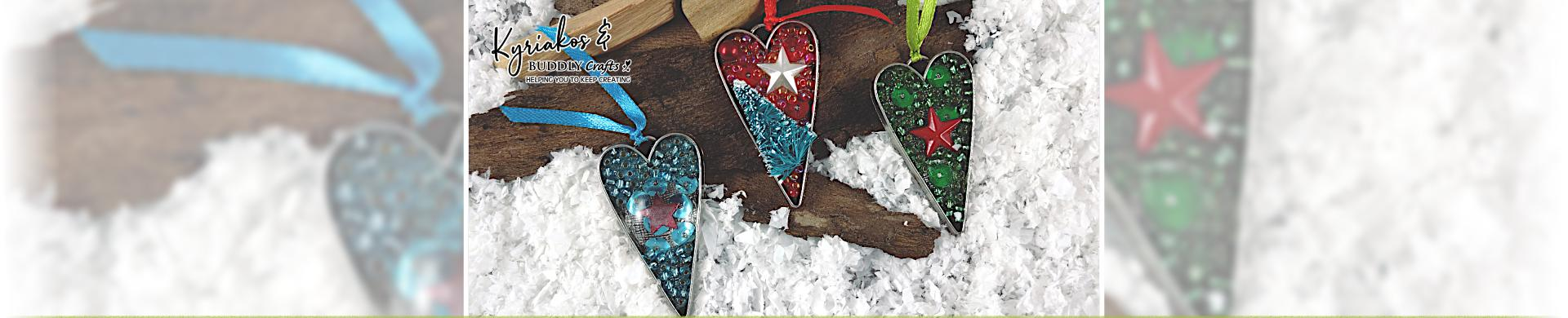# Metal Charms & Embellishments - Silver ToneCast and pressed metal charms, decorations and essential findings for mixed media arts.
• Brand
1. \$2.69
2. \$1.92
3. \$1.92
4. \$1.92
5. \$1.92
6. \$1.92
7. \$3.85
8. \$2.40
9. \$3.85
10. \$3.85
11. \$1.43
12. \$1.43
13. \$2.40
14. \$1.92
15. \$1.43
16. \$1.92
17. \$1.92
18. \$1.92
19. \$1.92
20. \$1.43
21. \$1.24
22. \$0.96
23. \$1.05
24. \$0.96
25. \$1.15
26. \$0.96
27. \$0.96
28. \$1.43
29. \$0.96
30. \$0.96
31. \$0.96
32. \$3.85
33. \$2.88
34. \$1.92
35. \$1.92
36. \$1.92
37. \$1.24
38. \$1.92
39. \$1.92
40. \$2.21
41. \$2.69
42. \$2.69
43. \$2.50
44. \$2.50